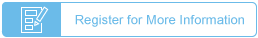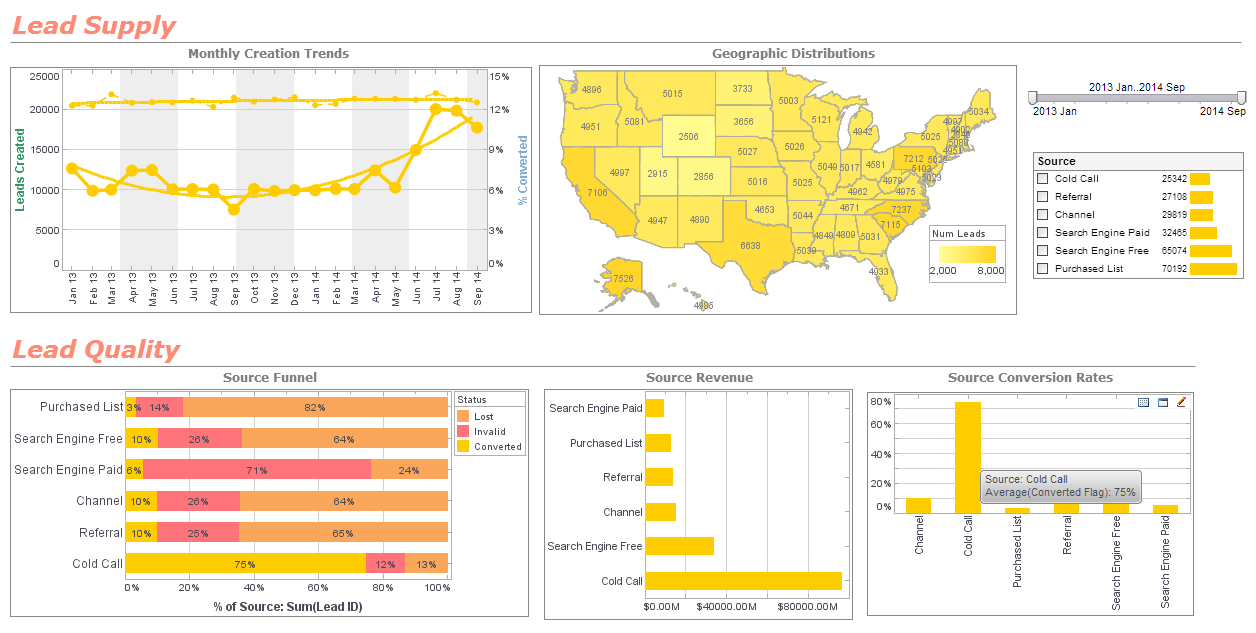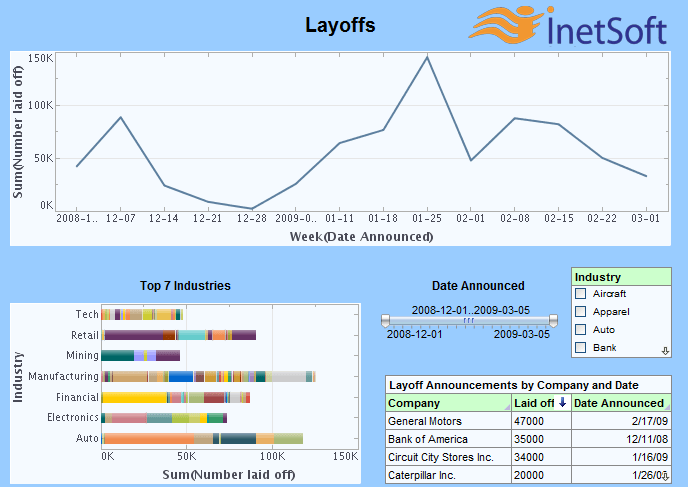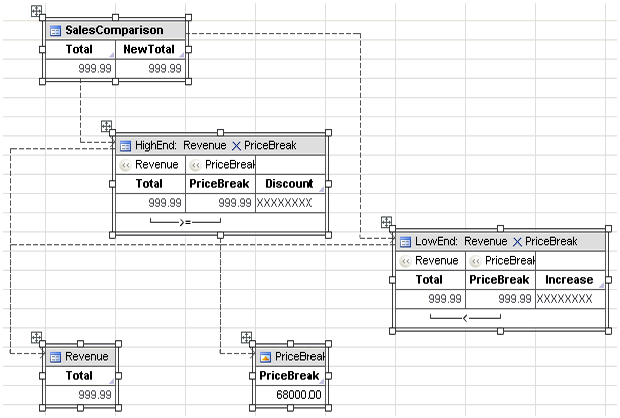# InetSoft Product Information: What-If Analysis

InetSoft's what-if analysis feature assists analysts in quantifying uncertainty in causal relationships and optimizing resource allocation while guiding decisions. InetSoft's Style Intelligence is the comprehensive real-time analytical reporting and dashboard software solution used at thousands of enterprises worldwide. View the example below to learn more about the Style Intelligence solution.

What-if analysis is the exploration of cause and effect relationships in data. This is one of the greatest strengths of the Data Worksheet, making use of the following features:

• Embedded tables

• Cell references in formulas

• Dynamic table updating

Suppose that all of our big customers have been requesting discounts, and we therefore decide to give a 5% discount on all large orders. Since we need to recover the lost revenue incurred by this discount, we will simultaneously need to increase the sales total on all smaller orders by 5%.

To implement this discount plan, we need to decide where to place the boundary between “large order” and “small order” so as to maintain the company's total revenue. This 'price break' value can be discovered through what-if analysis.View a 2-minute demonstration of InetSoft's easy, agile, and robust BI software.

In the example below, we will make use of the following components and methods:

• Embedded Table

• Joined Table

• Concatenated Table

• Group and aggregate options

• Expression fields

Let us assume we have a Worksheet table called 'Revenue'. The 'Revenue' table includes the fields 'Order Num' and 'Product Total', listing the total value of each order. Follow the steps below to analyze this data and detect the proper 'price break' threshold for classifying orders as “large” or “small”:

1. Create a new Embedded Table with one data cell, and name this table 'PriceBreak'. It will contain the 'price break' value that we experiment with. In the table cell, enter 40000 as the first guess for the price break value. This indicates that all orders with a total of \$40,000 qualify as “large orders”, and all orders with a total equal to or less than \$40,000 qualify as “small orders”.

Next, we will calculate the total sales of all of the large or 'High End' orders, and the total sales of all the small or 'Low End' orders, both before and after the discounts/increases are taken into account. We do this in order to compare the sales revenue before and after the discount model is put into effect.

2. In the 'Revenue' table, group the data by 'Order Num' and summarize by 'Product Total' so that we have the total sales amount for each order.

The data in the 'Revenue' table can now be split into two separate tables, 'HighEnd' and 'LowEnd'.

3. To obtain the 'HighEnd' table, specify a join between the 'Product Total' column and the 'PriceBreak' table, and set the join type to: Revenue.Total >= PriceBreak.PriceBreak

4. To obtain the 'LowEnd' table, specify a join between 'Product Total' and 'PriceBreak' and set the join type to: Revenue.Total < PriceBreak.PriceBreak

5. For the 'HighEnd' table, create an expression field named 'Discount' to calculate the new order total with the 5% discount applied. The SQL formula for the 'Discount' expression field should be set to
field['Total']*0.956. For the 'LowEnd' table, create an expression field named 'Increase' to calculate the new order total with the 5% increase applied. The SQL formula for the 'Increase' expression field should be set to field['Total']*1.05

Now that we have the new sales total values for the large and small orders, we can analyze the benefits of the current 'price break' value by comparing the difference between the original sales total and the new sales total.

7. Create a union of the 'HighEnd' table and the 'LowEnd' table, and name this Concatenated Table 'SalesComparison'. Name the right column 'NewTotal', because it contains the totals with discounts and increases applied.

8. Apply summarization on the 'Total' and 'NewTotal' fields of the 'SalesComparison' table to produce the final total revenue numbers.

Now every time we change the 'price break' value in the 'PriceBreak' embedded table, the values in the 'SalesComparison' table are automatically recalculated. After experimenting with a few numbers, we learn that \$50,000 is the optimal 'price break' for applying the new discount model without affecting the total company revenue.

In addition to performing what-if experimentation by entering values manually into an embedded table, you can also adjust table values graphically by using a Viewsheet input element (slider, spinner, etc.).

 Previous: Dashboard Conference Mailing